## Easily count unique and distinct values – 7 examples

Normally, in Excel, the unique values are the values that appear only once in the list without any duplications, and distinct values are all the different values (unique values + 1st duplicate occurrences). When working on a large dataset, you may need to count the number of unique and distinct values among duplicates from a list of cells as below screenshot shown. This tutorial will introduce some quick tricks for counting the unique and distinct values in Excel.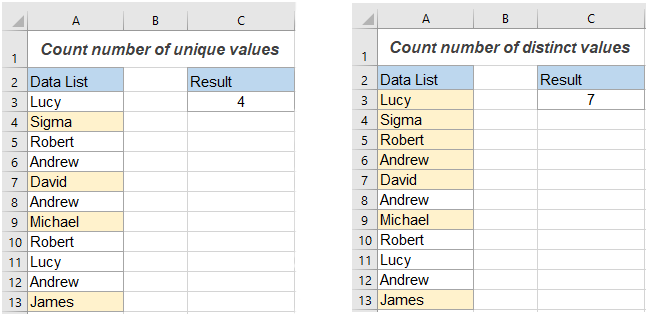Count unique values in Excel

Count distinct values (unique and 1st duplicate occurrences) in Excel

#### Count unique values in Excel

This section will talk about some formula examples for counting the number of unique values, including texts and numbers in a list.

#### Count unique values with formulas

Supposing, I have a list of names which contains some duplicate names within the name list. Now, I need to get the number of unique names only (which are filled with yellow color) as below screenshot shown: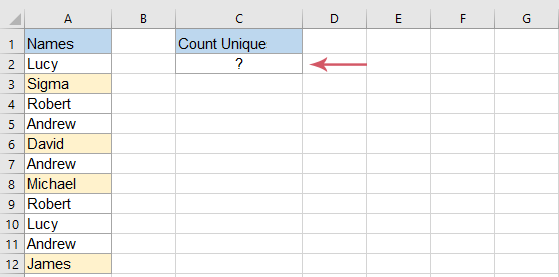To solve this issue, the following array formula can do you a favor:

Step 1: Input formula

Enter or copy the below formula into a blank cell where you want to output the result:

``=SUM(IF(COUNTIF(A2:A12,A2:A12)=1,1,0))``
Note: In the above formula, A2:A12 is the data list that you want to count unique.

Step 2: Press Ctrl + Shift + Enter keys to get the correct result: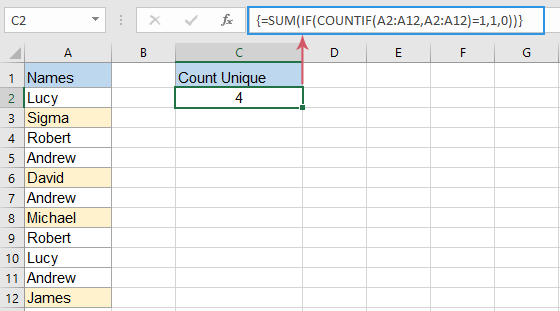Tips:

1. If your list of cells contains some other types of data, such as numbers, Booleans, error values, etc., when applying the above formula, it will get the number of unique values regardless of the data type.
2. When the data list contains blank cells, this formula will exclude the blank cells.
3. To count only the unique text values if there are other type of data, please apply the below array formula, and then press Ctrl + Shift + Enter keys to return the result:
``=SUM(IF(ISTEXT(A2:A12)*COUNTIF(A2:A12,A2:A12)=1,1,0))``
4. To count unique numbers only from a list of cells which contains any type of data, please use the following array formula, and then press Ctrl + Shift + Enter keys to return the result:
``=SUM(IF(ISNUMBER(A2:A12)*COUNTIF(A2:A12,A2:A12)=1,1,0))``

#### Count unique values with few clicks by Kutools for Excel

It may be painful for you to remember the formulas when you need to apply them next time. But, if you have Kutools for Excel, with its Count Unique Values option of Formulas Helper, you can get the result with only a few clicks. See below demo:

1. Click a cell to output the result;
2. Enable this feature by clicking Kutools > Formula Helper > Formula Helper > Statistical > Count unique values;
3. Select the list of data > OK.
Tips:
1. To apply this feature, you should download and install it firstly.
2. The Formulas Helper feature collects 40+ common used formulas,such as Extract unique values, Extract cells with unique values(include the first duplicates), Count the number of values separated by comma, Sum based on same text, and more...

#### Count unique values in Excel 365/2021 with formulas

If you are using Excel 365 or Excel 2021, there is a new UNIQUE function which can help you to create more simper formulas to count unique values in a set of data.

For example, to count the number of unique names in the range of A2:A12, please enter the following formula：

Step 1: Copy or enter the below formula

``=IFERROR(ROWS(UNIQUE(A2:A12,,TRUE)), 0)``
Note: In this formula, A2:A12 is the data list that you want to count unique values.

Step 2: Press Enter key to get the result: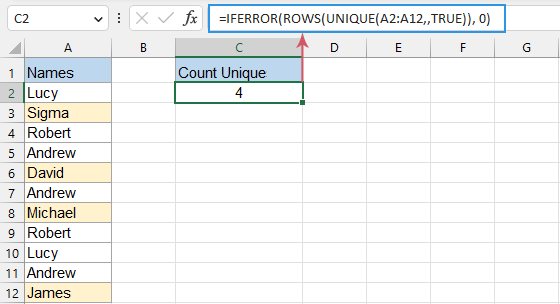Tips:

1. If the data list contains other types of data, such as text, numbers, Booleans, error values, etc., this formula will get the number of unique values regardless of the data type.
2. The above formula will count unique values including blank cells, if you want to exclude the blanks, please apply the below formula, and just need to press Enter key:
``=SUM(IF(COUNTIF(A2:A12,A2:A12)=1,1,0))``

#### Count distinct values (unique and 1st duplicate occurrences) in Excel

To count the different values (unique values and 1st duplicate occurrences) in a list of cells, here, I will introduce other formulas to achieve this task.

#### Count distinct values with formulas

In Excel, you can apply any one of below formulas to return the number of distinct values.

Step 1: Enter any one of the below formulas

Formula 1: After inputting the formula, press Enter key.

``=SUMPRODUCT(1/COUNTIF(A2:A12,A2:A12))      ``

Formula 2: After inputting the formula, press Ctrl + Shift + Enter keys.

``=SUM(1/COUNTIF(A2:A12,A2:A12))             ``
Note: In these formulas, A2:A12 is the data list that you want to count different values.

Result: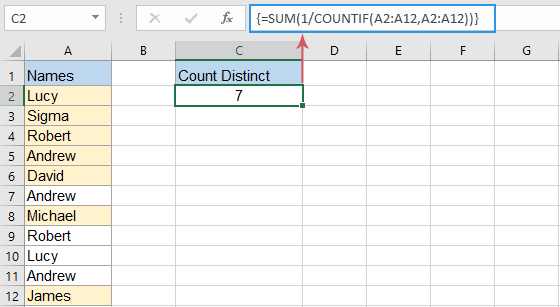Tips:

1. If the data list contains other types of data, such as numbers, Booleans, error values, etc., this formula will get the distinct values regardless the data type.
2. When using the above formula, you will get #DIV/0 error value if the data list contains blank cells. To fix and ignore blank cells, you should apply one of the below formulas:
Formula 1: After inputing the formula, press Enter key.
``=SUMPRODUCT((A2:A12<>"")/COUNTIF(A2:A12,A2:A12&""))        ``
Formula 2: After inputing the formula, press Ctrl + Shift + Enter keys.
``=SUM(IF(A2:A12<>"",1/COUNTIF(A2:A12, A2:A12), 0))       ``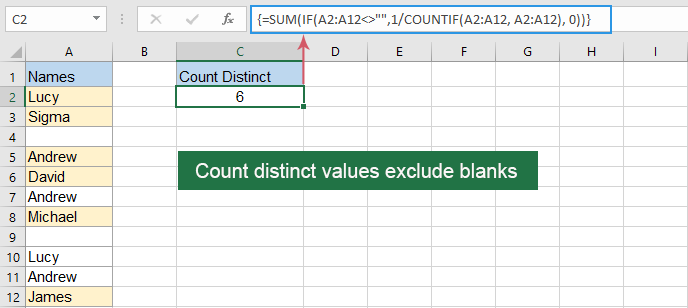3. To get the number of distinct text values only within a list of data, please apply the below array formula, and press Ctrl + Shift + Enter keys to get the result:
``=SUM(IF(ISTEXT(A2:A12),1/COUNTIF(A2:A12, A2:A12),""))``
4. To count only distinct numeric values, please use the following array formula, and press Ctrl + Shift + Enter keys to get the result:
``=SUM(IF(ISNUMBER(A2:A12),1/COUNTIF(A2:A12, A2:A12),""))``

#### Count distinct values with a few clicks by Kutools for Excel

If you need to apply the formulas frequently in your workbook, it may be painful for you to remember the formulas when you need to apply them next time. But, if you have Kutools for Excel, with its Count cells with unique values (include the first duplicate) option of Formulas Helper, you can get the result with only several clicks. See below demo:

1. Click a cell to output the result;
2. Enable this feature by clicking Kutools > Formula Helper > Statistical > Count unique cells with unique values (include the first duplicate);
3. Select the list of data > OK.
Tips:
1. To apply this feature, you should download and install it firstly.
2. The Formulas Helper feature collects 40+ common used formulas,such as Extract unique values, Extract cells with unique values(include the first duplicates), Count the number of values separated by comma, Sum based on same text, and more...

#### Count distinct values with PivotTable

In Excel, the PivotTable also can help to get the number of distinct values from a list of data, please do with the following steps:

Step 1: Create a pivot table

1. Select the data list, and then click Insert > PivotTable from the ribbon, see screenshot: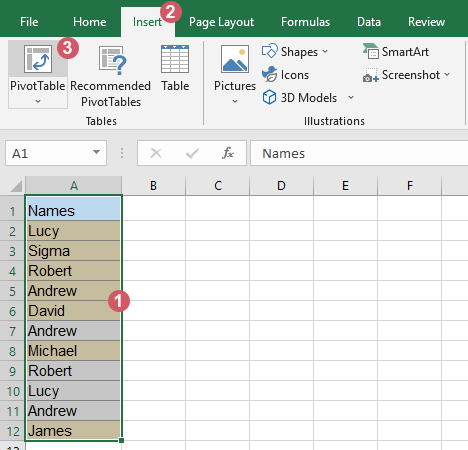2. In the popped out PivotTable from table or range dialog box:
(1). Choose a new worksheet or existing worksheet where you want to place the pivot table;
(2). Then check Add this data to the Data Model checkbox.
(3). Then click OK button.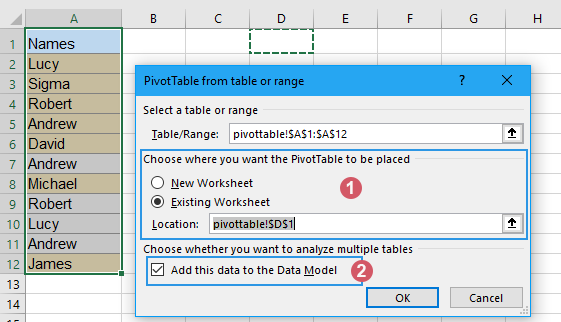Step 2: Arrange the field and choose Distinct Count option

1.  A PivotTable Fields pane is displayed, drag the Names field to Values area, see below demo:2. Then, click on the Count of Names drop down, and choose Value Field Settings, see screenshot:3. And then, a Value Field Settings dialog box will open, select Distinct Count from the list box under the Summarize Value By tab, and click OK button, see screenshot: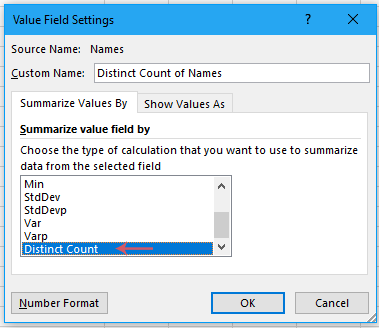Result:

Now, the created pivot table will display the distinct count of the data list as below screenshot shown: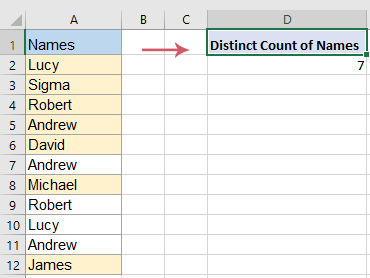Tips:

1. If you update your source data, to get the latest count, you just need to right click the pivot table and select Refresh option.
2. This Distinct Count option is only available in Excel 2013 and later.

#### Count distinct values in Excel 365/2021 with formulas

In Excel 365 or Excel 2021, you can use the new UNIQUE function with the normal COUNTA function to create an easy formula.

Please copy or enter the below formula into a blank cell, and press Enter key to get the result:

``=COUNTA(UNIQUE(A2:A12)) ``
Note: In this formula, A2:A12 is the data list that you want to count distinct values.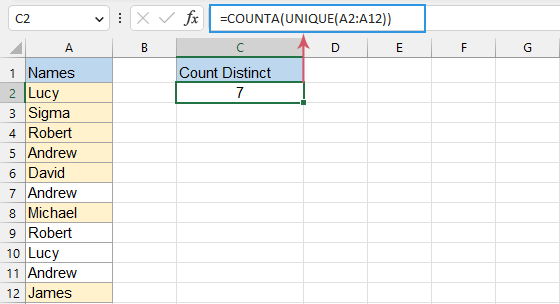Tips:

1. If the data list contains other types of data, such as text, numbers, Booleans, error values, etc., this formula will count the number of distinct values regardless of the data type.
2. The above formula will count distinct values including blank cells, if you want to exclude the blanks, please apply the below formula, and press Enter key to get the result:
``=COUNTA(UNIQUE(FILTER(A2:A12, A2:A12<>"")))    ``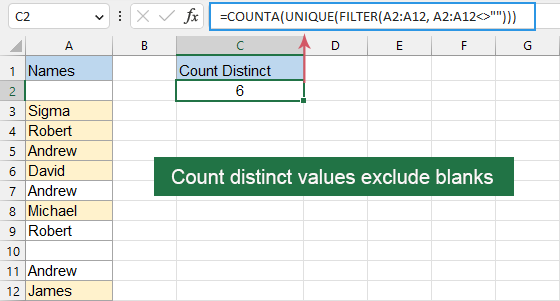#### Related Articles:

• Count unique values in pivot table
• By default, when we create a pivot table based on a range of data which contains some duplicate values, all the records will be counted as well, but, sometimes, we just want to count the unique values based on one column to get the right screenshot result. In this article, I will talk about how to count the unique values in pivot table.
• Count unique values in a filtered column
• If you have a list of names which populated with some duplicates in a filtered column, and now, you want to count the number of unique names only from this filtered list. Do you have any good solutions to quickly deal with this job in Excel?
• Count unique values with multiple criteria
• Here is a range of data containing several names, projects and locations, and what need to do is to find out the names which match the criteria I have list, and then count the each matched names once time only as below screenshot shown. Do you have any tricks on solving this job? In this article, I will introduce the tricks.
• Concatenate unique values
• If I have a long list of values which populated with some duplicate data, now, I want to find only the unique values and then concatenate them into a single cell. How could I deal with this problem quickly and easily in Excel?